# Python: Pandas, Working with DataFrames

Sure DataFrames look nice, but how can I work with them?

Let’s cover some basic tasks in pandas to get you started.

Let’s start by building a DataFrame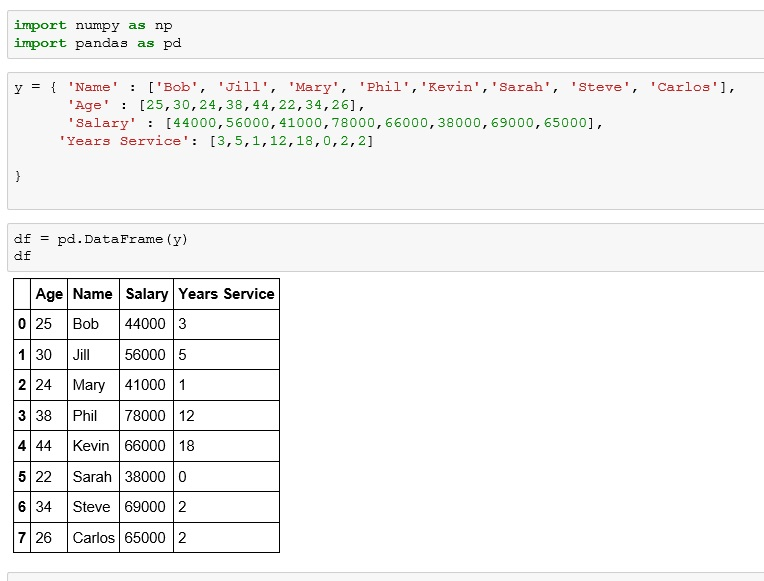I don’t like where they placed Age on my dataframe. I want to move it.

To do so, we are going to cover a couple of new terms: axis, drop() and insert()

### Axis

Using numpy and pandas, you will come across many functions that require you to enter an axis as a parameter. Axis 0 is your rows while Axis 1 is your columns. This is due to the way matrices are named with a 3×2 matrix having 3 rows and 2 columns and a 2×3 having 2 rows and 3 columns### drop()

To move the age column, I am first going to create a copy of my dataframe minus the age column. To do this, I am going to use the drop() function. The drop() function accepts two arguments drop(name, axis). In our case name = ‘Age’ and axis = 1 since we are referring to a column.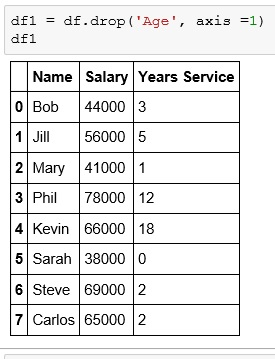### insert()

Now we want to insert the age column. The syntax for the insert() function is insert(insert point, name, data)Adding a new column is straight forward. Just DataFrame[new column name] = value.

Below I created at new column called ‘Age When Start’ that shows the age of employees when they started. I derived this value by subtracting Years Service column from Age column.## boolean column

You can create a boolean column using a boolean operator.## sort()

You can sort a dataframe by any column using sort_values()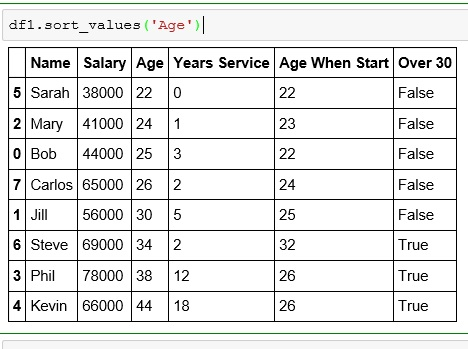Sort is set to ascending by default. To reverse it, set ascending = False

** remember in Python, True and False need to start with a capital letter.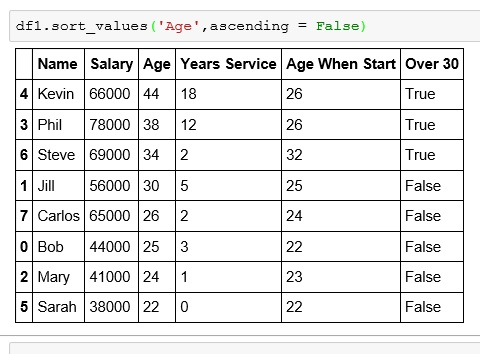## slicing

### by rows

slicing by rows is just like with a list### by columns

slicing by columns is a bit more complex. To slice by column name you have to use the dataframe.ix command.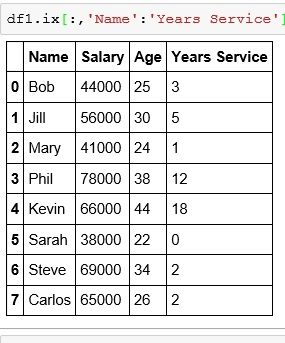If you enjoyed this lesson, click LIKE below, or even better, leave me a COMMENT.

Last Lesson: Pandas DataFrames

Next Lesson: Pandas: Rename a Column